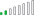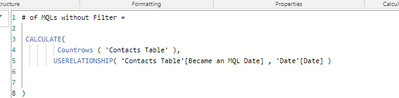cancel
Showing results for
Did you mean:Resolver II

## Userelationship with filter not displaying correctly

Hi all,

I have a simple table that has 2 columns with date, one called Create Date and the other called MQL Date. The Create Date has the active relationship to the Date table. See image #1.

I have two measures one with filter parameter, see image #2 and the other without filter parameter, see image #3.

The filter parameter filters for MQL Date after 1/1/2022. The outcome should be the same but it is not.

Why am I getting two different values. See image #4

image#1 - data modelimage #2 - with filterimage #3 - without filterimage#4 - output

Can someone help me see what I am doing wrong? Thanks in advance.https://1drv.ms/u/s!AlTfdvX0WzaQ118wqvrLVBtgeCMV?e=3T7xrd

1 ACCEPTED SOLUTIONResolver II

Below is the solution to the query. It is about filter and context transition.

Hello @ysherriff,

Thank You for posting your query onto the Forum.

The answer is - “No, the outcome will not be the same”.

The reason being is, two different contexts are applied inside the “CALCULATE()” function i.e., “FILTER()” which is an iterator function and when there’s an iteration, “CALCULATE()” executes a context transition. And secondly, there’s a “USERELATIONSHIP()” function which is a context modifier.

So the way execution happens in your measure i.e., in “# of MQL with Filter” is in the following order -

CALCULATE starts from the current context

1). Then it starts to “evaluate” the filter arguments i.e., both row and filter context (In this case, “FILTER()” function since it’s an iterator and check the logic row-by-row).

2). Since it’s iterating row-by-row, “context transition” is bound to happen. That is, adds all the column filter to the new context (In this case, “‘Contacts Table’[Became an MQL Date]).

3). Now, it starts to evaluate the context modifiers (In this case, “USERELATIONSHIP()” function).

4). And then lastly, applies the filter arguments which are evaluated in “Step No. 1”.

In a simple way, here’s how the engine evaluates the measure - "# of MQL with Filter" at the back-end -

```# of MQL with Filter =
COUNTROWS(
CALCULATETABLE(
'Contacts Table' ,
FILTER( 'Contacts Table' ,
'Contacts Table'[Became an MQL Date] >= DATE( 2022, 1 , 1 ) ) ,
USERELATIONSHIP( 'Contacts Table'[Became an MQL Date] , 'Date'[Date] ) ) )```

So first, it creates a table i.e., “Contacts Table” then it starts the evaluation of the filter argument i.e., “FILTER()” function which iterates the logic row-by-row over the table where MQL Date >= 01/01/2022. Once the iteration over that column is done, it performs the context transition where that column “MQL Date” is added into a new context. Until now, the no. of rows generated is still 74.

Now, modifier starts working i.e., “USERELATIONSHIP()” function. And this is where, results starts to change. It creates the relationship with the “‘Date’[Date]” and converts the results of the “MQL Date” which is stored in a new context. So now, any rows prior to the condition i.e., 01/01/2022 is removed entirely from the “Contacts Table”. These are the rows which it removes from the table. Below is the screenshot provided for the reference -

Rows Removed During The Context Transition1539×265 27 KB

And lastly, again applies the fiter arguments which were mentioned at the beginning i.e., in Step No. 1. (If you create a physical table with the DAX formula provided above by removing the “COUNTROWS()” function, you’ll see that the number of rows generated is 67 and not 74. And then if you check all the columns which contains the date information, you’ll observe that all the information will be available only from or after 01/01/2022 and not prior to that. That’s because, MQL Date was evaluated in a new context based on the logic and created a table where all information is only available after that date/logic. Below is the screenshot provided for the reference -)

Rows Generated At Back-End During Context Transition2238×1202 337 KB

Whereas in the measure - “# of MQLs without Filter”, “CALCULATE()” is only modifying the context i.e., “USERELATIONSHIP()” is simply changing the relationship from “Create Date” to “Become an MQL Date” by retaining all the rows of the table i.e., No Context Transistion Is Performed since there’s no iteration.

So at the back-end this is how engine evaluates the measure by introducing “ALL()” on the “Become an MQL Date” column -

```# of MQLs without Filter =
CALCULATE( COUNTROWS( 'Contacts Table' ) ,
FILTER( ALL( 'Contacts Table'[Became an MQL Date] ) ,
'Contacts Table'[Became an MQL Date] >= DATE( 2022 , 1 , 1 ) ) ,
USERELATIONSHIP( 'Contacts Table'[Became an MQL Date] , 'Date'[Date] ) )```

You can cross-verify it by creating a table with the below provided DAX formula in order to check the number of rows generated at the back-end. See this -

```Table =
CALCULATETABLE(
'Contacts Table' ,
FILTER( ALL( 'Contacts Table'[Became an MQL Date] ) ,
'Contacts Table'[Became an MQL Date] >= DATE( 2022, 1 , 1 ) ) ,
USERELATIONSHIP( 'Contacts Table'[Became an MQL Date] , 'Date'[Date] ) )```

Rows Generated At Back-End When Only Context Modifier Is In Place2239×1198 346 KB

As you can observe in the above screenshot, in this case, it includes the information prior to the date - 01/01/2022 in the other date related columns.

Hoping you find this explanation useful and helps you in understanding how CALCULATE executes the orders and most importantly in which order.

Important Note: While cross-verifying the results, I’ve applied a filter on the column “Become an MQL Date” where the range is between “01/01/2022 - 31/01/2022” since that is the same range, you’ve selected in your slicer onto the report page So in case, if you want to cross-verify it, apply the same range and then check the results.

Thanks and Warm Regards,
Harsh

2 REPLIES 2Resolver II

Below is the solution to the query. It is about filter and context transition.

Hello @ysherriff,

Thank You for posting your query onto the Forum.

The answer is - “No, the outcome will not be the same”.

The reason being is, two different contexts are applied inside the “CALCULATE()” function i.e., “FILTER()” which is an iterator function and when there’s an iteration, “CALCULATE()” executes a context transition. And secondly, there’s a “USERELATIONSHIP()” function which is a context modifier.

So the way execution happens in your measure i.e., in “# of MQL with Filter” is in the following order -

CALCULATE starts from the current context

1). Then it starts to “evaluate” the filter arguments i.e., both row and filter context (In this case, “FILTER()” function since it’s an iterator and check the logic row-by-row).

2). Since it’s iterating row-by-row, “context transition” is bound to happen. That is, adds all the column filter to the new context (In this case, “‘Contacts Table’[Became an MQL Date]).

3). Now, it starts to evaluate the context modifiers (In this case, “USERELATIONSHIP()” function).

4). And then lastly, applies the filter arguments which are evaluated in “Step No. 1”.

In a simple way, here’s how the engine evaluates the measure - "# of MQL with Filter" at the back-end -

```# of MQL with Filter =
COUNTROWS(
CALCULATETABLE(
'Contacts Table' ,
FILTER( 'Contacts Table' ,
'Contacts Table'[Became an MQL Date] >= DATE( 2022, 1 , 1 ) ) ,
USERELATIONSHIP( 'Contacts Table'[Became an MQL Date] , 'Date'[Date] ) ) )```

So first, it creates a table i.e., “Contacts Table” then it starts the evaluation of the filter argument i.e., “FILTER()” function which iterates the logic row-by-row over the table where MQL Date >= 01/01/2022. Once the iteration over that column is done, it performs the context transition where that column “MQL Date” is added into a new context. Until now, the no. of rows generated is still 74.

Now, modifier starts working i.e., “USERELATIONSHIP()” function. And this is where, results starts to change. It creates the relationship with the “‘Date’[Date]” and converts the results of the “MQL Date” which is stored in a new context. So now, any rows prior to the condition i.e., 01/01/2022 is removed entirely from the “Contacts Table”. These are the rows which it removes from the table. Below is the screenshot provided for the reference -

Rows Removed During The Context Transition1539×265 27 KB

And lastly, again applies the fiter arguments which were mentioned at the beginning i.e., in Step No. 1. (If you create a physical table with the DAX formula provided above by removing the “COUNTROWS()” function, you’ll see that the number of rows generated is 67 and not 74. And then if you check all the columns which contains the date information, you’ll observe that all the information will be available only from or after 01/01/2022 and not prior to that. That’s because, MQL Date was evaluated in a new context based on the logic and created a table where all information is only available after that date/logic. Below is the screenshot provided for the reference -)

Rows Generated At Back-End During Context Transition2238×1202 337 KB

Whereas in the measure - “# of MQLs without Filter”, “CALCULATE()” is only modifying the context i.e., “USERELATIONSHIP()” is simply changing the relationship from “Create Date” to “Become an MQL Date” by retaining all the rows of the table i.e., No Context Transistion Is Performed since there’s no iteration.

So at the back-end this is how engine evaluates the measure by introducing “ALL()” on the “Become an MQL Date” column -

```# of MQLs without Filter =
CALCULATE( COUNTROWS( 'Contacts Table' ) ,
FILTER( ALL( 'Contacts Table'[Became an MQL Date] ) ,
'Contacts Table'[Became an MQL Date] >= DATE( 2022 , 1 , 1 ) ) ,
USERELATIONSHIP( 'Contacts Table'[Became an MQL Date] , 'Date'[Date] ) )```

You can cross-verify it by creating a table with the below provided DAX formula in order to check the number of rows generated at the back-end. See this -

```Table =
CALCULATETABLE(
'Contacts Table' ,
FILTER( ALL( 'Contacts Table'[Became an MQL Date] ) ,
'Contacts Table'[Became an MQL Date] >= DATE( 2022, 1 , 1 ) ) ,
USERELATIONSHIP( 'Contacts Table'[Became an MQL Date] , 'Date'[Date] ) )```

Rows Generated At Back-End When Only Context Modifier Is In Place2239×1198 346 KB

As you can observe in the above screenshot, in this case, it includes the information prior to the date - 01/01/2022 in the other date related columns.

Hoping you find this explanation useful and helps you in understanding how CALCULATE executes the orders and most importantly in which order.

Important Note: While cross-verifying the results, I’ve applied a filter on the column “Become an MQL Date” where the range is between “01/01/2022 - 31/01/2022” since that is the same range, you’ve selected in your slicer onto the report page So in case, if you want to cross-verify it, apply the same range and then check the results.

Thanks and Warm Regards,
HarshCommunity Champion

because you have a slicer also filtering the dates only between 1/1/2011 and 1/31/2022. try to change the slicer covering the date before 1/1/2022, then you shall see the difference.